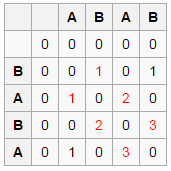# Longest Common Substring problem

The longest common substring problem is the problem of finding the longest string (or strings) that is a substring (or are substrings) of two strings.

The problem differs from problem of finding longest common subsequence. Unlike subsequences, substrings are required to occupy consecutive positions within the original sequences.

For example, the longest common substring of the strings ‘ABABC’, ‘BABCA’ is string ‘BABC’ having length 4. Other common substrings are ‘ABC’, ‘A’, ‘AB’, ‘B’, ‘BA’, ‘BC’ and ‘C’.

Naive solution would be to consider all substrings of the second string and find the longest substring that is also a substring of first string. The time complexity of this solution would be O((m+n)*m2) as it takes (m+n) time for substring search and there are m2 substrings of second string. We can optimize this method by considering substrings in order of their decreasing lengths and return as soon any substring matches the first string. But worst case time complexity still remains the same when no common characters are present.

##### Can we do better?

The idea is to find the longest common suffix for all pairs of prefixes of the strings using Dynamic Programming using the relation –

LCSuffix[i][j] = | LCSuffix[i-1][j-1] + 1      (if X[i-1] = Y[j-1])
| 0                           (otherwise)

Here,
0 <= i - 1 < m       where m is the length of the string X
0 <= j - 1 < n       where n is the length of the string Y

For example, consider strings ‘ABAB’ and ‘BABA’.Finally, the length of the longest common substring would be the maximal of these longest common suffixes of all possible prefixes.

Below solution finds the length of longest repeated Subsequence of sequences X and Y iteratively by using optimal substructure property of LCS problem.

## C++

Output:

The Longest common substring is AB

## Java

Output:

Length of Longest Common Substring is 4

The time complexity of above solution is O(n2) and auxiliary space used by the program is O(n2). The space complexity of above solution can be improved to O(n) as calculating LCS of a row of the LCS table requires only the solutions to the current row and the previous row. We can also store only non-zero values in the rows. This can be done using hash tables instead of arrays.

We can also solve this problem in O(m + n) time by using generalized suffix tree. We will be soon discussing suffix tree approach in a separate post.

Exercise: Write space optimized code for iterative version.(8 votes, average: 4.25 out of 5)Loading...

Please use our online compiler to post code in comments. To contribute, get in touch with us.
Like us? Please spread the word and help us grow. Happy coding 🙂Subscribe
Notify ofGuest
jay prakash

Is there any recursive method for it, not necessary optimized, just a slow recursive function?GuestGuest

@kishore i was asking about recursive function for printing the LCS not to the length of max common substring.Guest
Hitman47

refer for O(n) space solution of longest common sub-string.
refer: https://ideone.com/tfhY6PGuest

there is some problem i see in this if u can explain plz
1- the function return string and in the output u say it will return the length
2- the code will return sub continues string for example S1=”ABDC” S2=”ABC” the code will return AB not ABC if that what u want i did not understand that from the explanation before the link
3- great subject plz continueGuest

Hi, your code seems to be working fine, but in your last example (at least when executed in Java), your output is different than what you’d get when you execute it. It seems to be correct for the c++ version, but the Java should also return the same result: “The Longest common substring is AB”.Guest
anonymous

If you have two strings such as “DABCD” and “BABCA”, for which the substring would be “ABC”, your program prints out “AB” because it doesn’t keep track of the beginning of the substring.Guest
Vishist Varugeese

It prints “AB” because the input is fixed in the code. Change it to your input before running the code. It works fine.